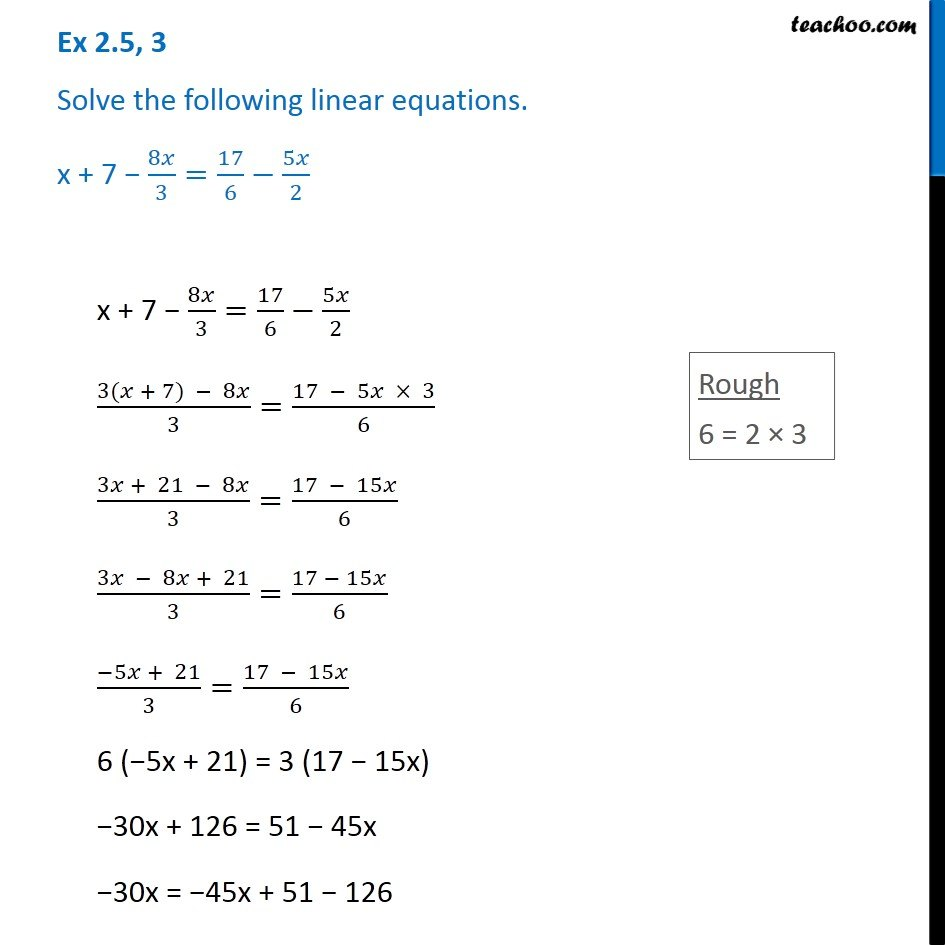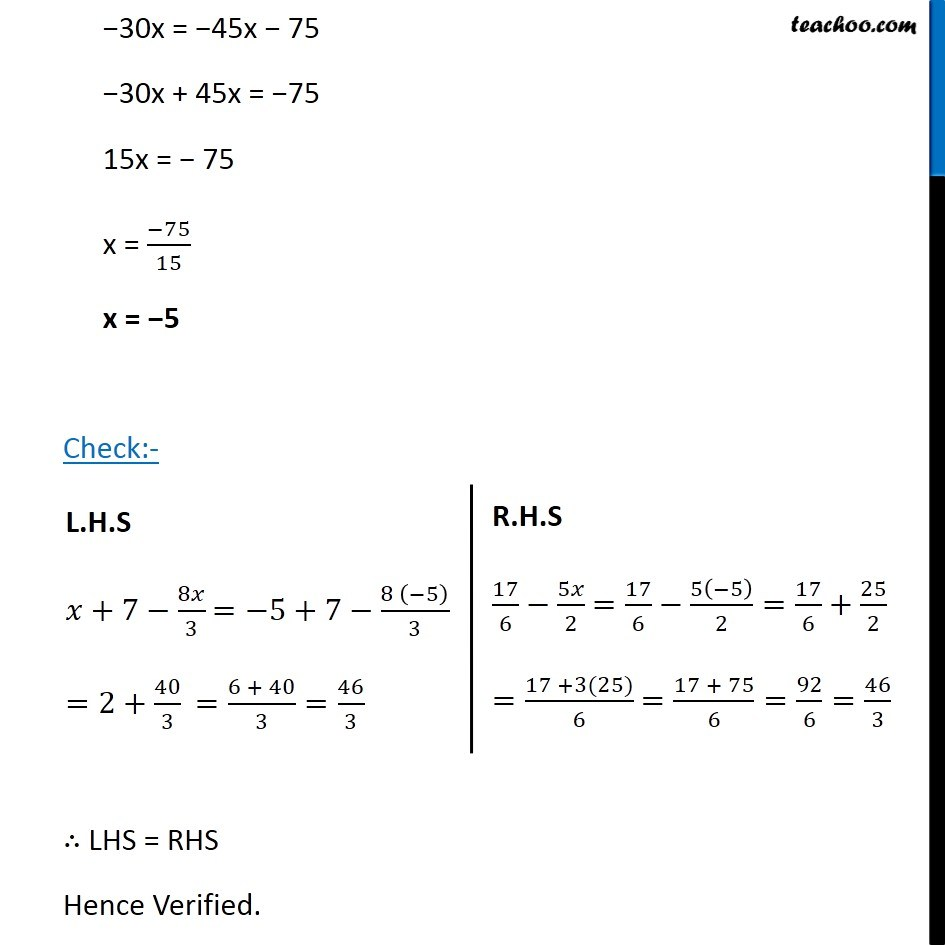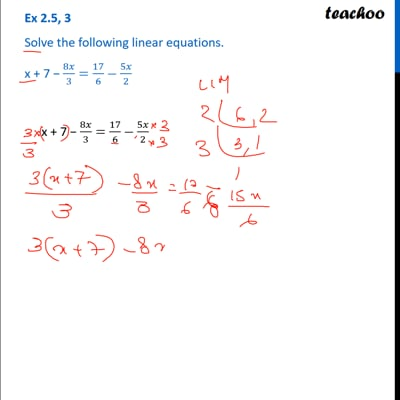Ex 2.5

Chapter 2 Class 8 Linear Equations in One Variable
Serial order wiseThis video is only available for Teachoo black users

Get live Maths 1-on-1 Classs - Class 6 to 12

### Transcript

Ex 2.5, 3 Solve the following linear equations. x + 7 − 8𝑥/3=17/6−5𝑥/2x + 7 − 8𝑥/3=17/6−5𝑥/2 (3(𝑥 + 7) − 8𝑥)/3=(17 − 5𝑥 × 3)/6 (3𝑥 + 21 − 8𝑥)/3=(17 − 15𝑥)/6 (3𝑥 − 8𝑥 + 21)/3=(17 − 15𝑥)/6 (−5𝑥 + 21)/3=(17 − 15𝑥)/6 6 (−5x + 21) = 3 (17 − 15x) −30x + 126 = 51 − 45x −30x = −45x + 51 − 126 −30x = −45x − 75 −30x + 45x = −75 15x = − 75 x = (−75)/15 x = −5 Check:- ∴ LHS = RHS Hence Verified. L.H.S 𝑥+7−8𝑥/3 =−5+7−(8 (−5))/3 =2+40/3 =(6 + 40)/3=46/3 R.H.S 17/6−5𝑥/2 =17/6−5(−5)/2=17/6+25/2 =(17 +3(25))/6 =(17 + 75)/6=92/6=46/3